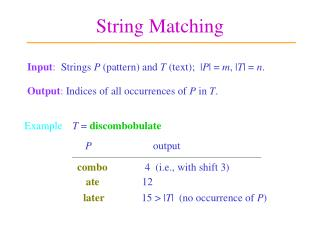DownloadDownload PresentationString Matching

# String Matching

Télécharger la présentation## String Matching

- - - - - - - - - - - - - - - - - - - - - - - - - - - E N D - - - - - - - - - - - - - - - - - - - - - - - - - - -
##### Presentation Transcript

1. String Matching Input:Strings P (pattern) and T (text); |P| = m, |T| = n. Output: Indices of all occurrences of P in T. Example T =discombobulate P output combo4 (i.e., with shift 3) ate12 later15 > |T| (no occurrence of P)

2. Applications Text retrieval Computational biology - DNA is a one-dimensional (1-D) string of characters A’s, G’s, C’s, T’s. - All information for 3-D protein folding is contained in protein sequence itself and independent of the environment. Searching for DNA patterns Comparing two or more DNA strings for similarities Reconstructing DNA strings from overlapping fragments.

3. Sliding the Pattern Template T =b i o l o g yP =l o g i c n = 7 m = 5 b i o l o g y l o g i c b i o l o g y l o g i c b i o l o g y l o g i c T  P No match! b i o l o g y l o g i c b i o l o g y l o g i c T = P, T = P, T = P, but T  P T  P b i o l o g y l o g i c b i o l o g y l o g i c T  P

4. Another Example T =b i o l o g i c a lP =l o g i c n = 10 m = 5 b i o l o g i c a l l o g i c Match found! return 4.

5. The Naive Matcher Pattern: P[1..m] Text: T[1..n] Naive-String-Matcher(T, P) // find all occurrences of P in T. fors = 1 ton  m +1 do ifP[1 .. m] = T[s .. s+m1] then print “Pattern occurs at index” s T: s s+m-1 P: 1 m

6. P T 1 2 3 n m+1 n Time Complexity m(n  m + 1) comparisons (as below) in the worst case. m chars n  m + 1 blocks, each requiring m comparisons Time complexity isO(mn)!

7. Example a input a b b 0 1 0 0 1 state a 1 0 0 transition function b Finite Automaton Afinite automatonconsists of a finite setQof states a start state a set A of accepting states a finite input alphabet  a transition function d: Q    Q. accepting state start state

8. Always begins at the start state. Accepts a string if it ends at an accepting state after accepting all string chars. Otherwise, it rejects the string. a b 0 1 a b Accepting a String input state sequence accepts? Yes aabba 010001 No bbabb 000100

9. input state a b P b 1 0 a b 0 1 2 0 a a b a a 0 1 2 3 4 2 2 3 b a b 3 4 0 a a 2 0 4 b state sequence A String Matching Automaton Ex. Pattern P =a a b a aba not rescanned due to transition 42 T = a b b a a a b a a b a Pattern occurs at indices 5 and 8! 0 1 0 0 1 2 2 3 4 2 3 4

10. Key Ideas of Automaton Matching Slide pattern forward by more than one position if possible. Do not rescan chars of T that have already been examined.

11. 3 But computing d requiresO(m ||)!// details omitted. The Automaton Matcher Finite-Automaton-Matcher(T, d, m) n = length[T] q = 0 // current state fori = 1 ton do q = d(q, T[i]) // d function precomputed if q = m// match succeeds then print “Pattern occurs at index” i m+1 O(n)if the state transition function d is available.